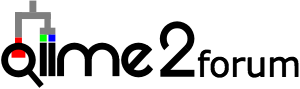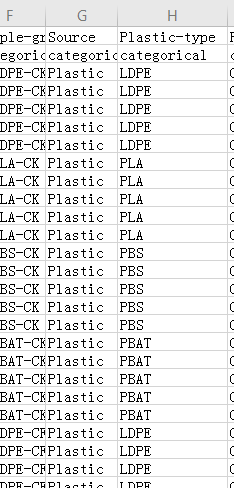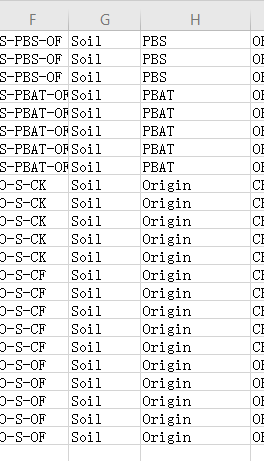# How to make a heatmap use some groups of all samples in qiime2R?

Dear all,
now I want to make a heatmap use some groups of all samples in qiime2R. But, I can’t get a filterd taxonomy.qza by metadata. So,how can I use the command to select some samples to make a garph? For example,I have the "Source"and “Plastic” two column in my metadata.
And I dont want the the samples of “soil” in the “Source”,and “origin” in “Plastic” displayed in my graph. How should I do?``````    setwd(dir="D:/Rdata/micro_plastic_2020/16s/try1")
library(tidyverse)
library(qiime2R)

SVs<-apply(SVs, 2, function(x) x/sum(x)*100) #convert to percent
SVsToPlot<-
data.frame(MeanAbundance=rowMeans(SVs)) %>% #find the average abundance of a SV
rownames_to_column("Feature.ID") %>%
arrange(desc(MeanAbundance)) %>%
top_n(30, MeanAbundance) %>%
pull(Feature.ID) #extract only the names from the table

SVs %>%
as.data.frame() %>%
rownames_to_column("Feature.ID") %>%
gather(-Feature.ID, key="SampleID", value="Abundance") %>%
mutate(Feature.ID=if_else(Feature.ID %in% SVsToPlot,  Feature.ID, "Remainder")) %>% #flag features to be collapsed
group_by(SampleID, Feature.ID) %>%
summarize(Abundance=sum(Abundance)) %>%
mutate(NormAbundance=log10(Abundance+0.01)) %>% # do a log10 transformation after adding a 0.01% pseudocount. Could also add 1 read before transformation to percent
left_join(taxonomy) %>%
mutate(Feature=paste(Feature.ID, Taxon)) %>%
mutate(Feature=gsub("[kpcofgs]__", "", Feature)) %>% # trim out leading text from taxonomy string

mutate(`Fertilizer-type`=factor(`Fertilizer-type`, levels=c("CK","CF","OF"))) %>%

ggplot(aes(x=`Fertilizer-type`, y=Feature, fill=NormAbundance)) +
geom_tile() +
facet_grid(~`Plastic-type`, scales="free_x") +
theme_q2r() +
theme(axis.text.x=element_text(angle=45, hjust=1)) +
scale_fill_viridis_c(name="log10(% Abundance)")
ggsave("qiime2/image/heatmap.pdf", height=4, width=11, device="pdf")
``````

Q2：How can I set the level of taxonomy ？